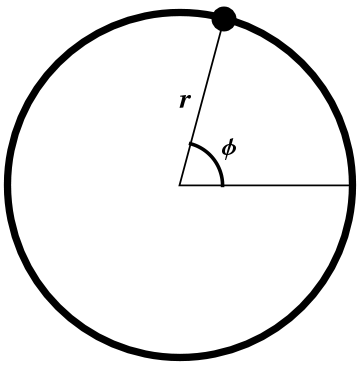Polar coordinates are useful for situations with circular symmetry in the plane. The polar coordinates ($$r\text{,}$$$$\phi$$) of a point $$P$$ are given by the distance $$r$$ of $$P$$ from the origin and the angle $$\phi$$ from the positive $$x$$-axis to $$P\text{,}$$ as shown in Figure 1.1.1.Figure 1.1.1. The construction of the polar coordinates ($$r\text{,}$$$$\phi$$) at an arbitrary point.
Notation: When we think of the plane as a cross-section of spherical coordinates, we will use the pair ($$r\text{,}$$$$\phi$$) for polar coordinates. When we think of the plane as a cross-section of cylindricals coordinates, we will use the pair ($$s\text{,}$$$$\phi$$) for polar coordinates. In other references, you may also see the angle called $$\theta$$ instead of $$\phi\text{;}$$ we use $$\phi$$ to agree with our conventions for (cylindrical and) spherical coordinates.
It is important to remember that the angle $$\phi$$ does not measure distance; it has the wrong dimensions. Angles (in radians) are defined as the ratio of arclength to radius on the circle, so the arclength from the positive $$x$$-axis to $$P$$ along the circle shown in the figure is $$r\phi\text{.}$$